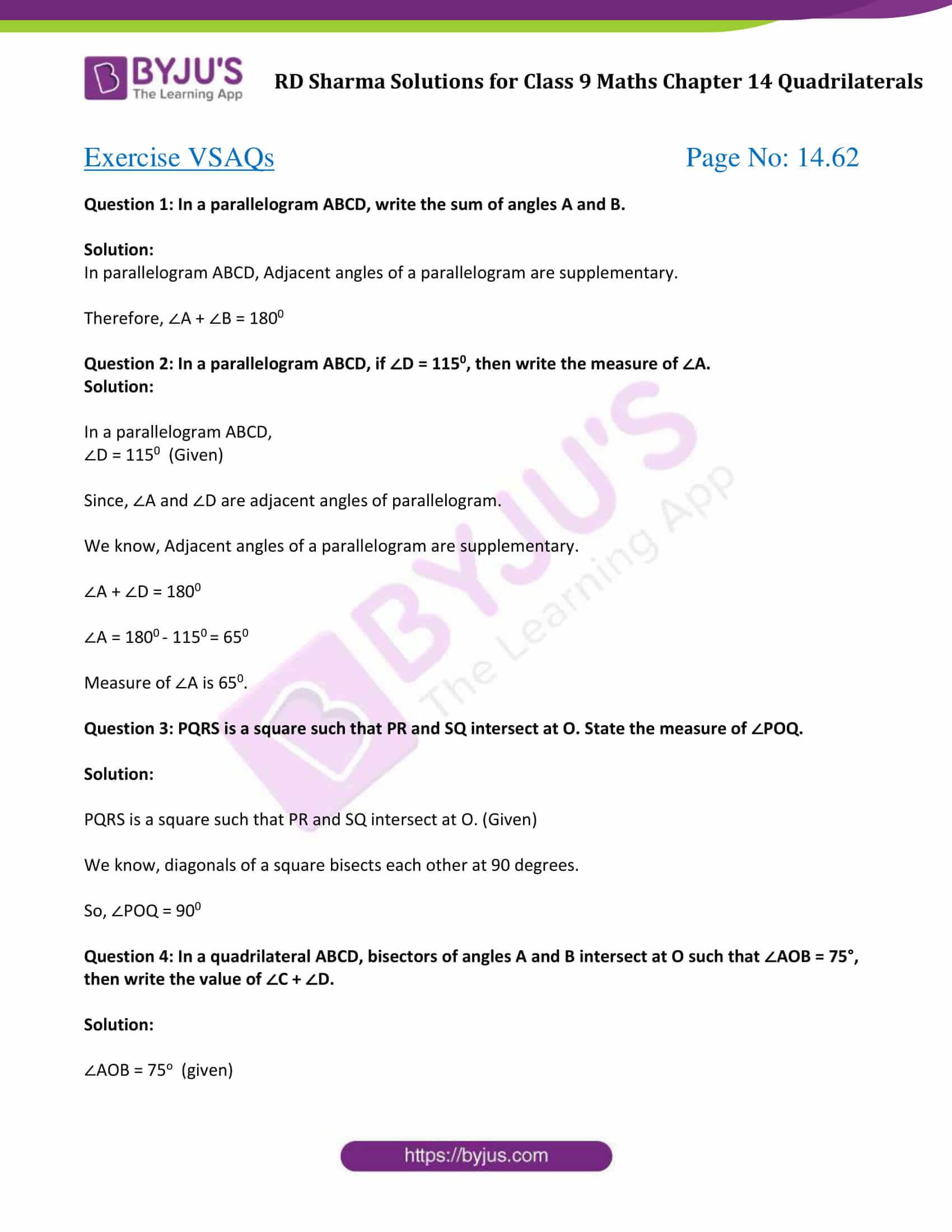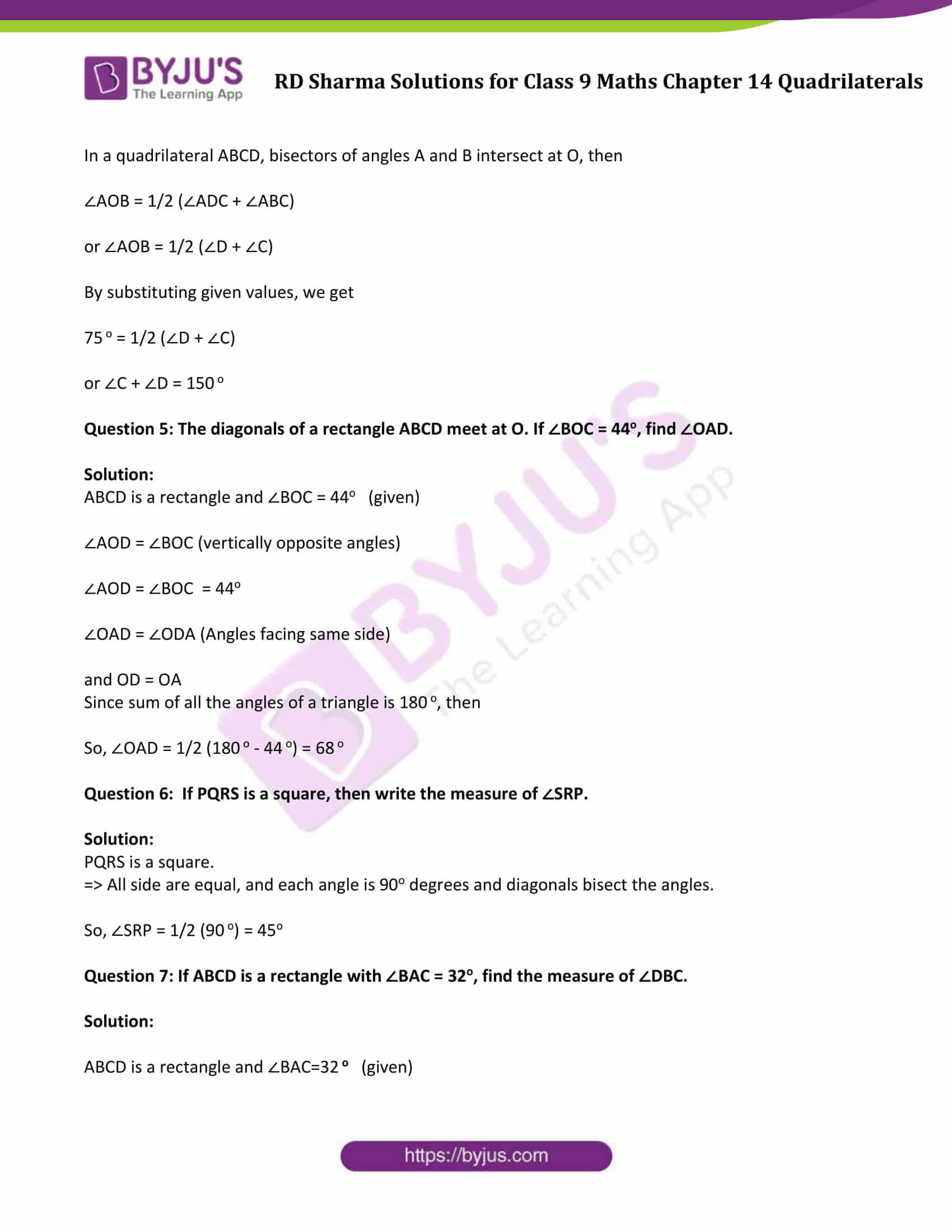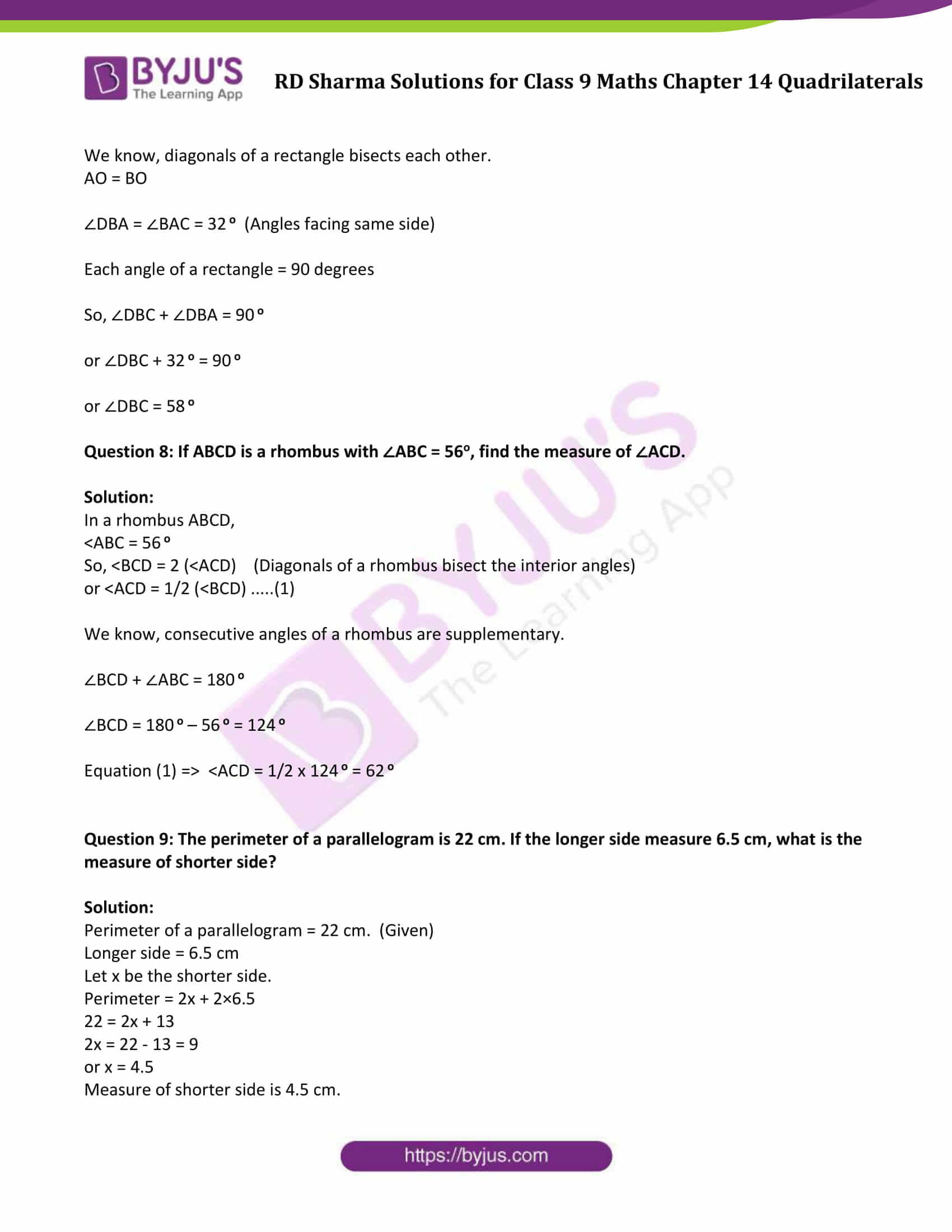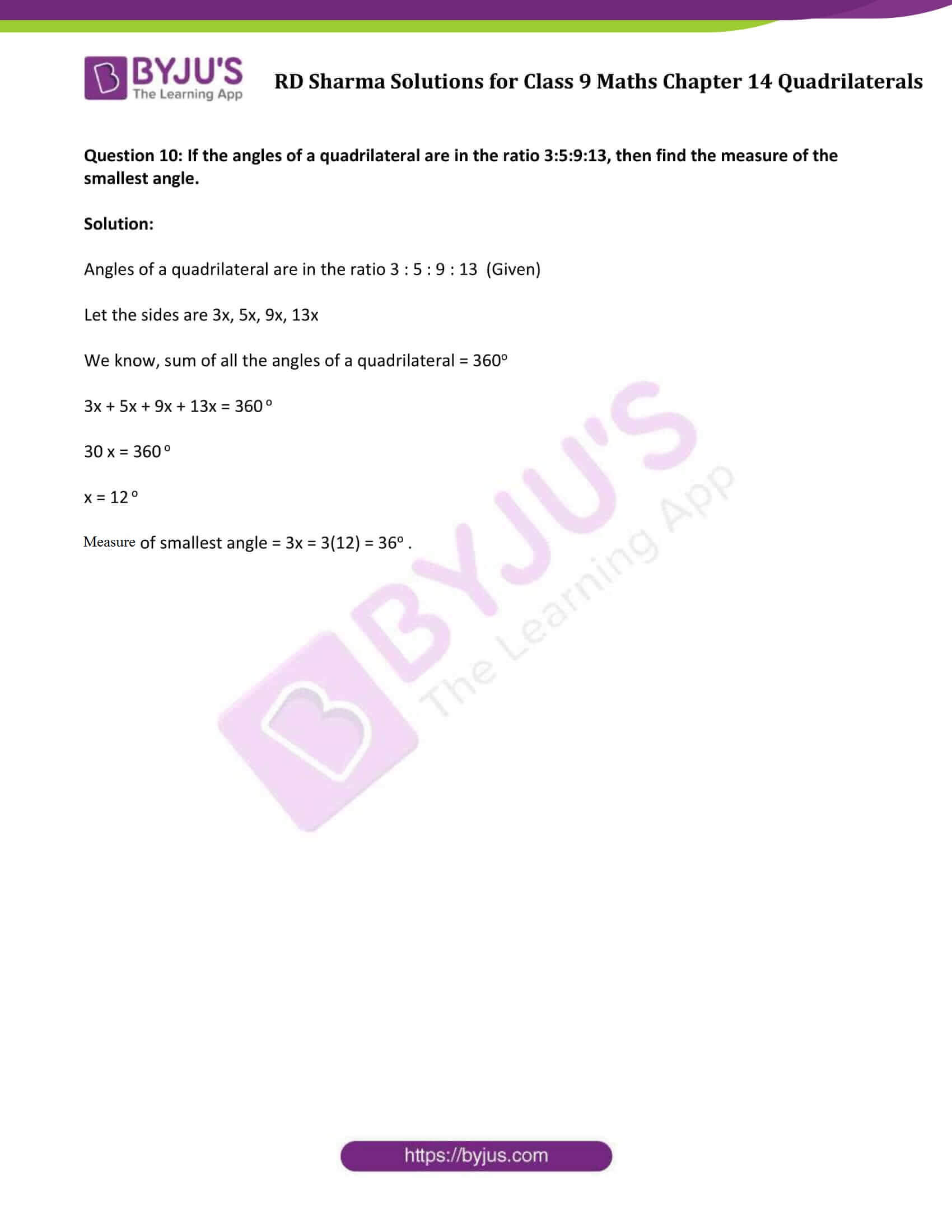# RD Sharma Solutions for Class 9 Maths Chapter 14 Quadrilaterals Exercise VSAQs

RD Sharma class 9 Mathematics chapter 14 exercise VSAQs solutions for Quadrilaterals are provided here. In this exercise, students will revise their concepts studied in this chapter on Quadrilaterals. Thus, here we have our set of RD Sharma solutions for class 9 to help students in their endeavor to have a better understanding of Quadrilaterals.### Access Answers to Maths RD Sharma Solutions for Class 9 Chapter 14 Quadrilaterals Exercise VSAQs Page number 14.62

Question 1: In a parallelogram ABCD, write the sum of angles A and B.

Solution:

In parallelogram ABCD, Adjacent angles of a parallelogram are supplementary.

Therefore, ∠A + ∠B = 1800

Question 2: In a parallelogram ABCD, if ∠D = 1150, then write the measure of ∠A.

Solution:

In a parallelogram ABCD,

∠D = 1150 (Given)

Since, ∠A and ∠D are adjacent angles of parallelogram.

We know, Adjacent angles of a parallelogram are supplementary.

∠A + ∠D = 1800

∠A = 1800 – 1150 = 650

Measure of ∠A is 650.

Question 3: PQRS is a square such that PR and SQ intersect at O. State the measure of ∠POQ.

Solution:

PQRS is a square such that PR and SQ intersect at O. (Given)

We know, diagonals of a square bisects each other at 90 degrees.

So, ∠POQ = 900

Question 4: In a quadrilateral ABCD, bisectors of angles A and B intersect at O such that ∠AOB = 75°, then write the value of ∠C + ∠D.

Solution:

∠AOB = 75o (given)

In a quadrilateral ABCD, bisectors of angles A and B intersect at O, then

∠AOB = 1/2 (∠ADC + ∠ABC)

or ∠AOB = 1/2 (∠D + ∠C)

By substituting given values, we get

75 o = 1/2 (∠D + ∠C)

or ∠C + ∠D = 150 o

Question 5: The diagonals of a rectangle ABCD meet at O. If ∠BOC = 44o, find ∠OAD.

Solution:

ABCD is a rectangle and ∠BOC = 44o (given)

∠AOD = ∠BOC (vertically opposite angles)

∠AOD = ∠BOC = 44o

∠OAD = ∠ODA (Angles facing same side)

and OD = OA

Since sum of all the angles of a triangle is 180 o, then

So, ∠OAD = 1/2 (180 o – 44 o) = 68 o

Question 6: If PQRS is a square, then write the measure of ∠SRP.

Solution:

PQRS is a square.

⇒ All side are equal, and each angle is 90o degrees and diagonals bisect the angles.

So, ∠SRP = 1/2 (90 o) = 45o

Question 7: If ABCD is a rectangle with ∠BAC = 32o, find the measure of ∠DBC.

Solution:

ABCD is a rectangle and ∠BAC=32 o (given)

We know, diagonals of a rectangle bisects each other.

AO = BO

∠DBA = ∠BAC = 32 o (Angles facing same side)

Each angle of a rectangle = 90 degrees

So, ∠DBC + ∠DBA = 90 o

or ∠DBC + 32 o = 90 o

or ∠DBC = 58 o

Question 8: If ABCD is a rhombus with ∠ABC = 56o, find the measure of ∠ACD.

Solution:

In a rhombus ABCD,

<ABC = 56 o

So, <BCD = 2 (<ACD) (Diagonals of a rhombus bisect the interior angles)

or <ACD = 1/2 (<BCD) …..(1)

We know, consecutive angles of a rhombus are supplementary.

∠BCD + ∠ABC = 180 o

∠BCD = 180 o – 56 o = 124 o

Equation (1) ⇒ <ACD = 1/2 x 124 o = 62 o

Question 9: The perimeter of a parallelogram is 22 cm. If the longer side measure 6.5 cm, what is the measure of shorter side?

Solution:

Perimeter of a parallelogram = 22 cm. (Given)

Longer side = 6.5 cm

Let x be the shorter side.

Perimeter = 2x + 2×6.5

22 = 2x + 13

2x = 22 – 13 = 9

or x = 4.5

Measure of shorter side is 4.5 cm.

Question 10: If the angles of a quadrilateral are in the ratio 3:5:9:13, then find the measure of the smallest angle.

Solution:

Angles of a quadrilateral are in the ratio 3 : 5 : 9 : 13 (Given)

Let the sides are 3x, 5x, 9x, 13x

We know, sum of all the angles of a quadrilateral = 360o

3x + 5x + 9x + 13x = 360 o

30 x = 360 o

x = 12 o

Measure of smallest angle = 3x = 3(12) = 36o .

## RD Sharma Solutions for Class 9 Maths Chapter 14 Quadrilaterals Exercise VSAQs

RD Sharma Solutions Class 9 Maths Chapter 14 Quadrilaterals Exercise VSAQs is based on quadrilaterals angles, different types of quadrilaterals, its special cases, and many more. This study material is designed by the BYJU’S subject experts to help students understand the concept easily. Download your pdf now and start practising to score good marks.